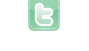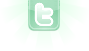This site requires JavaScript, please enable it in your browser!# ObjectCounter..

i have a class squirrel . i want to make a counter for object , when object created it should increase and object name will store as "s"+counter so i can differentiate object. i tried this but i know it's wrong
```public class squirrel extends Actor
{
int count=0;
String name;

public squirrel()
{
count++;
name="s"+count;
System.out.println(name);
GreenfootImage image = new  GreenfootImage(10, 10);
image.setColor(Color.RED);
image.fill();
setImage(image);
}
```
nicpatel wrote...
i have a class squirrel . i want to make a counter for object , when object created it should increase and object name will store as "s"+counter so i can differentiate object. i tried this but i know it's wrong << Code Omitted >>
As written, an int count field is given to each squirrel instance created with an initial value of zero (0). So, all squirrels will have a name value of "s0". What you need is a single field that all squirrels can use; namely, a class field. You can make the count field a class field by making it a static field. Change line 3 to:
`public static int count;`
You may want to zero the field in your world constructor before creating any squirrels as static fields only initialize during compilation (it will not initialize when you reset the scenario). You can use the following line to zero the field:
`squirrel.count = 0;`
Thanks a lot for previous help
```for(Object obj:getWorld().getObjects(squirrel.class))
{
int flag=0;

for(int i=0;i<100;i++)
{
if(matrix[i]==(((squirrel)obj).name))
{
matrix[i]=((Actor)obj).getX()+"";
matrix[i]=((Actor)obj).getY()+"";
flag=1;
break;
}
else{}
}
if(flag==0)
{
matrix[mp]=((squirrel)obj).name;
matrix[mp]=((Actor)obj).getX()+"";
matrix[mp]=((Actor)obj).getY()+"";
mp++;
}

}```
in this code , i m inserting name ,x-coordinate , y-coordinate for each squirrel object found . but problem is when i delete the object how can i remove entry of that object from matrix. if( which condition ) { } if you have any idea about condition on removing object
I think you can just change line 11 to the following:
`if (((Actor)obj).getWorld() == null) matrix[i] = ""; else flag = 1;`
(PREVIOUS HELP NOT WORKING ) SO I DID SOMETHING LIKE THIS IF YOU FOUND EFFICIENT WAY OR ANY OPTIMIZATION IN BELOW CODE PLEASE INFORM.
```          int c=0;
String[] temp=new String[getWorld().numberOfObjects()];
for(Object obj:getWorld().getObjects(squirrel.class))
{
temp[c]=((squirrel)obj).name;
c++;
}
for(int i=0;i<100;i++)
{
if(matrix[i]!=null)
{
for(int t=0;t<c;t++)
{
if(matrix[i]==temp[t])
{
System.out.println("Name:"+matrix[i]+"x:"+matrix[i]+"y:"+matrix[i]);
break;
}
else if(t==c-1 && matrix[i]!=temp[t])
{
for(int p=i;p<99;p++)
{
if(matrix[p]!=null && matrix[p+1]!=null)
{
matrix[p]=matrix[p+1];
matrix[p]=matrix[p+1];
matrix[p]=matrix[p+1];
}
else
{
matrix[p]="";
matrix[p]="";
matrix[p]="";
}
}
}
}

}
if(matrix[i+1]==null)
break;
}```
I do not know all the things you do with the matrix. The following would be an easy way to keep it up to date when an actor is removed.
```public void removeObject(Actor actor)
{
super.removeObject(actor)
if (actor instanceof squirrel)
{
squirrel sq = (squirrel)actor;
for (int i=0; i<100; i++) if (sq.name.equals(matrix[i]) matrix[i] = new String[] { "", "", "" }; // or = null;
}
}```
and printing the list would be quite simple:
```for (int i=0; i<100; i++)
{
if (!"".equals(matrix[i]) // or if (matrix[i] != null)
{
System.out.println("Name:"+matrix[i]+"x"+matrix[i]+"y"+matrix[i]);
}
}```
```if( object not moving)  <--can you help me here!
{

update(matrix);
}```
i want to update location matrix when object stop to move.... is it possible.
nicpatel wrote...
<< Code Omitted >> i want to update location matrix when object stop to move.... is it possible.
I think we will need to see more of the squirrel class code.
You need to login to post a reply.